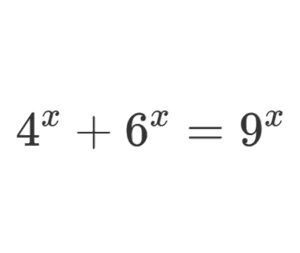Home -> Solved problems -> Solve for real x

## Solve the equation for real values of $$x$$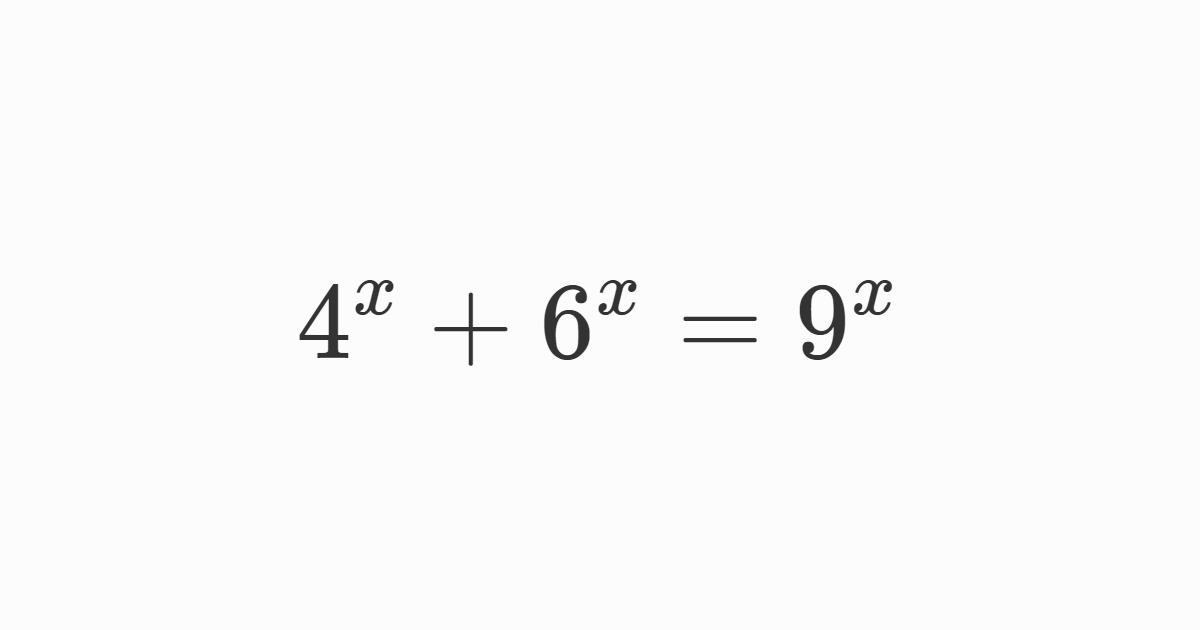### Solution

We are dealing with an equation with numbers to the power of $$x$$. Let’s start by dividing all the terms by $$4^{x}$$, we get $\frac{4^{x}}{4^{x}}+\frac{6^{x}}{4^{x}}=\frac{9^{x}}{4^{x}}$ $\Rightarrow1+\left(\frac{6}{4}\right)^{x}=\left(\frac{9}{4}\right)^{x}$ $\Rightarrow1+\left(\frac{3}{2}\right)^{x}=\left(\left(\frac{3}{2}\right)^{2}\right)^{x}$ $\Rightarrow1+\left(\frac{3}{2}\right)^{x}=\left(\frac{3}{2}\right)^{2x}$ To continue, we make a change of variables. Let $$u=\left(\frac{3}{2}\right)^{x}$$ thus $$\left(\frac{3}{2}\right)^{2x}=u^{2}$$  Now after making the changes, we get a quadratic equation of form $u^{2}=1+u$ $\Rightarrow u^{2}-u-1=0$ We calculate the discriminant $$\delta$$ $\delta=\left(-1\right)^{2}-4\left(1\right)\left(-1\right)$ $=1+4$ $=5$ $u=\frac{-\left(-1\right)-\sqrt{5}}{2\left(1\right)}$ $=\frac{1-\sqrt{5}}{2}$ $\color{red} {Or}$ $u=\frac{-\left(-1\right)+\sqrt{5}}{2\left(1\right)}$ $=\frac{1+\sqrt{5}}{2}$ $u=\left(\frac{3}{2}\right)^{x}>0$ Therefore the solution $$u=\frac{1-\sqrt{5}}{2}$$ is to be rejected. $\Rightarrow u=\frac{1+\sqrt{5}}{2}$ $\log u=\log\left(\left(\frac{3}{2}\right)^{x}\right)$ $=x\log\left(\frac{3}{2}\right)$ $\Rightarrow x=\frac{\log u}{\log\left(\frac{3}{2}\right)}$ $\Rightarrow x=\frac{\log \left(\frac{1+\sqrt{5}}{2}\right)}{\log\left(\frac{3}{2}\right)}\approx1.19$
Home -> Solved problems -> Solve for real x

### Related Topics

Find the volume of the square pyramid as a function of $$a$$ and $$H$$ by slicing method.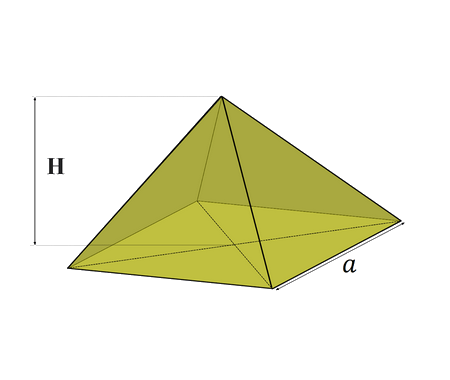Prove that $\lim_{x \rightarrow 0}\frac{\sin x}{x}=1$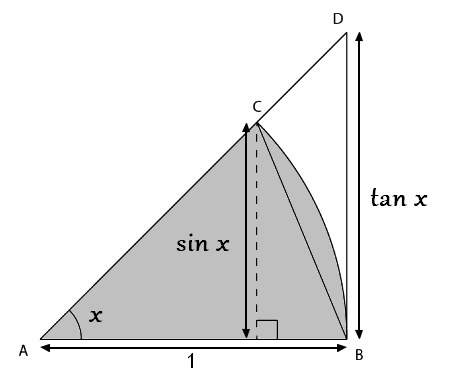Prove that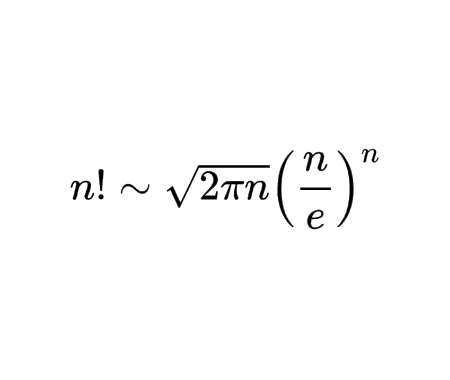Calculate the half derivative of $$x$$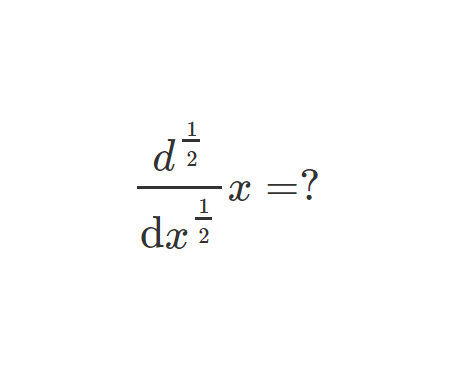Prove Wallis Product Using Integration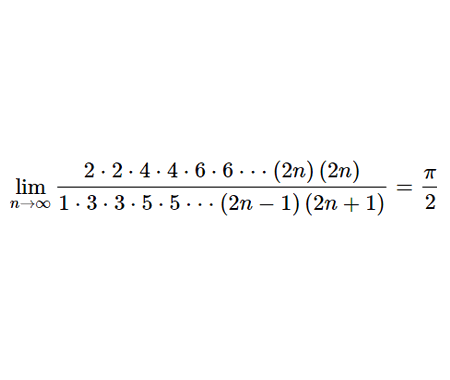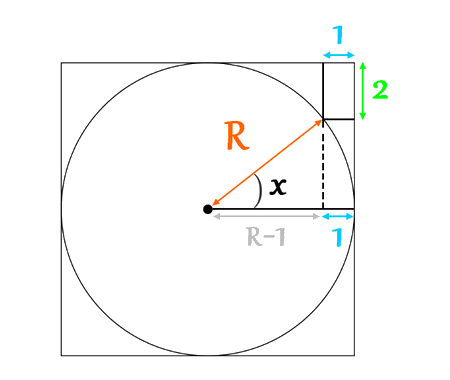Calculate the volume of Torus using cylindrical shells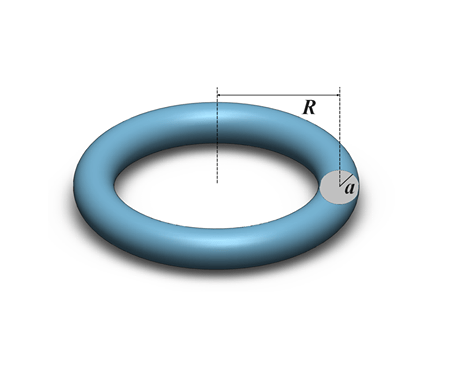Find the derivative of exponential $$x$$ from first principles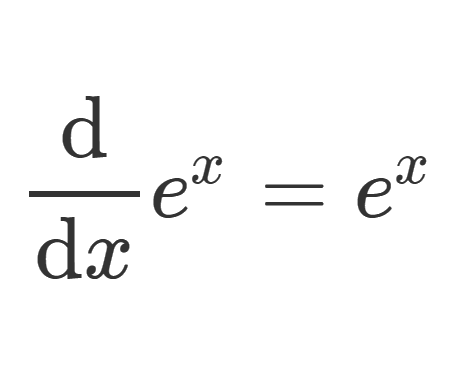Calculate the sum of areas of the three squares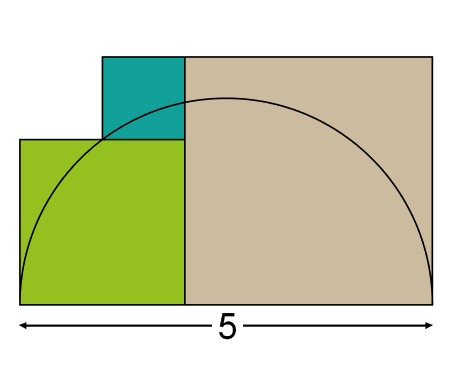Find the equation of the curve formed by a cable suspended between two points at the same height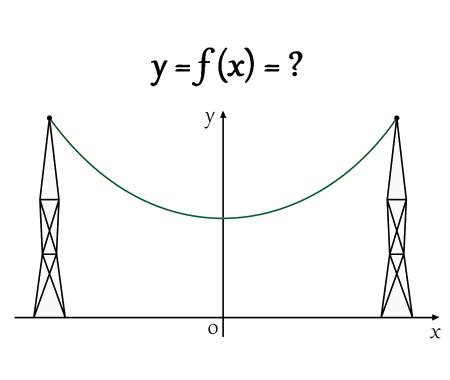Solve the equation for real values of $$x$$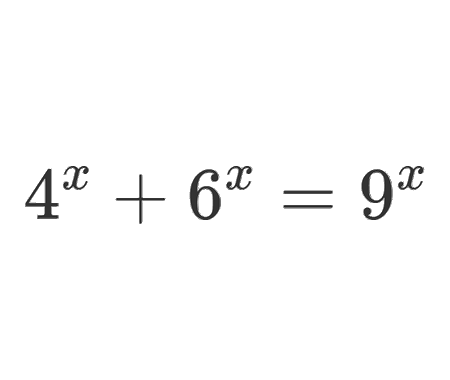Solve the equation for $$x\epsilon\mathbb{R}$$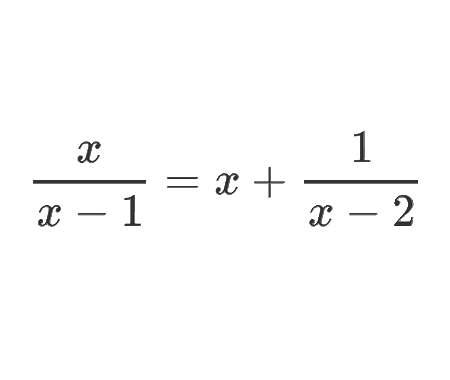Determine the angle $$x$$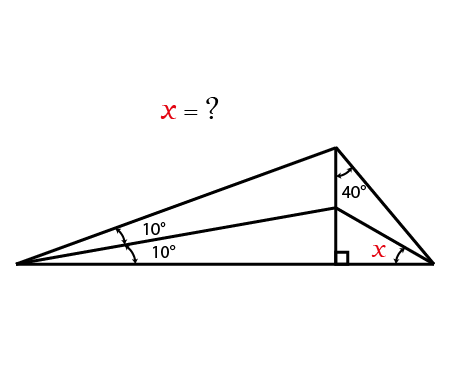Calculate the following limit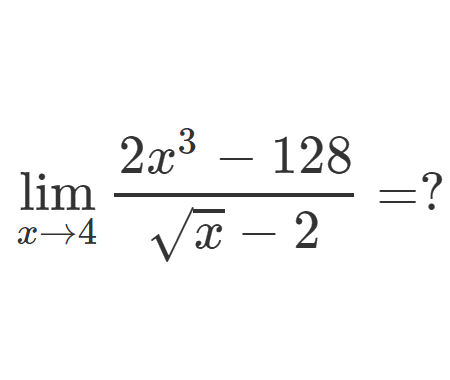Calculate the following limit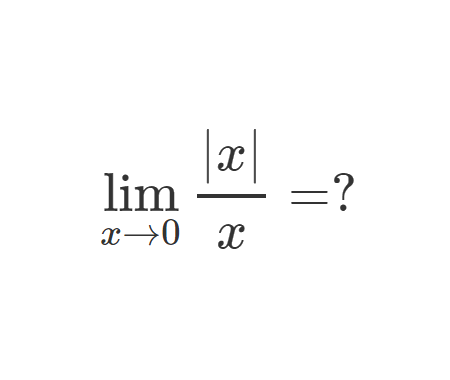Calculate the integral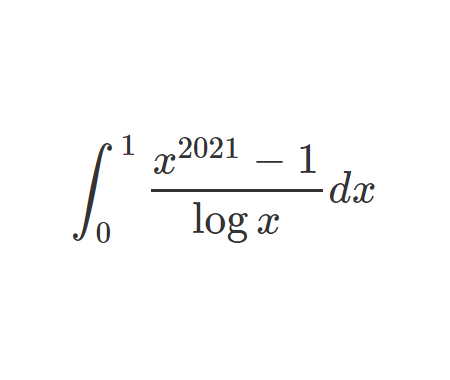Home -> Solved problems -> Solve for real x

#### Share the solution: Solve for real x